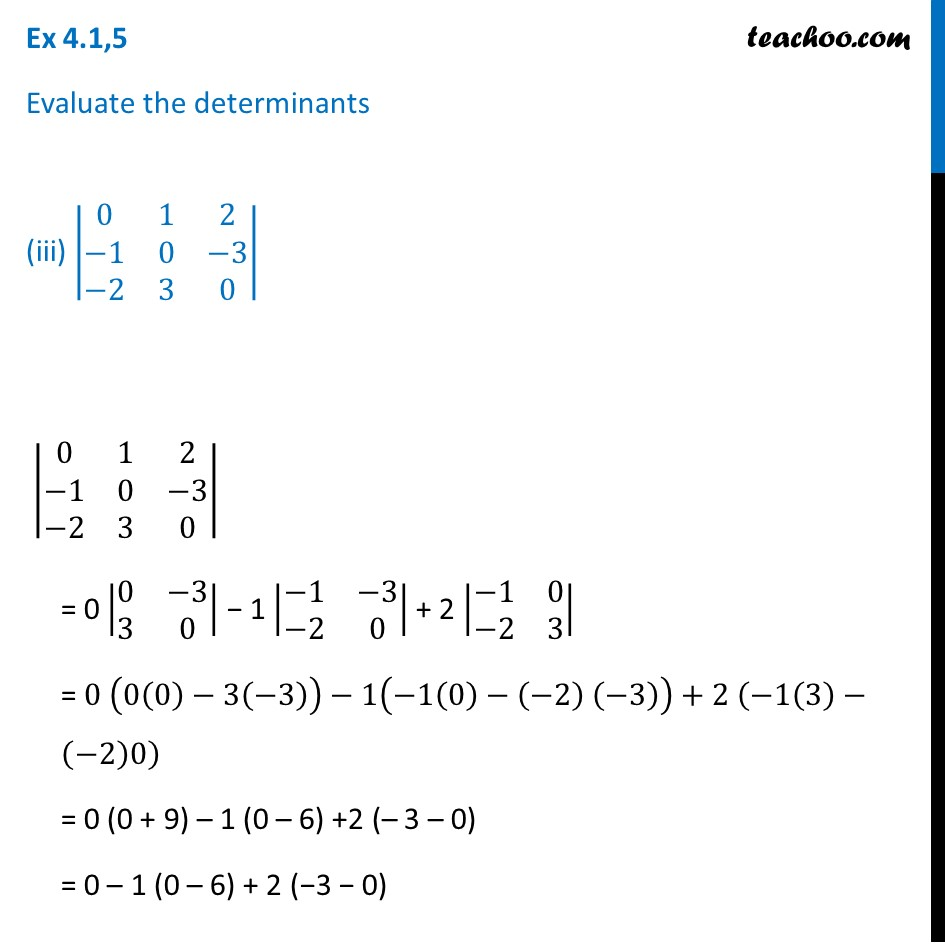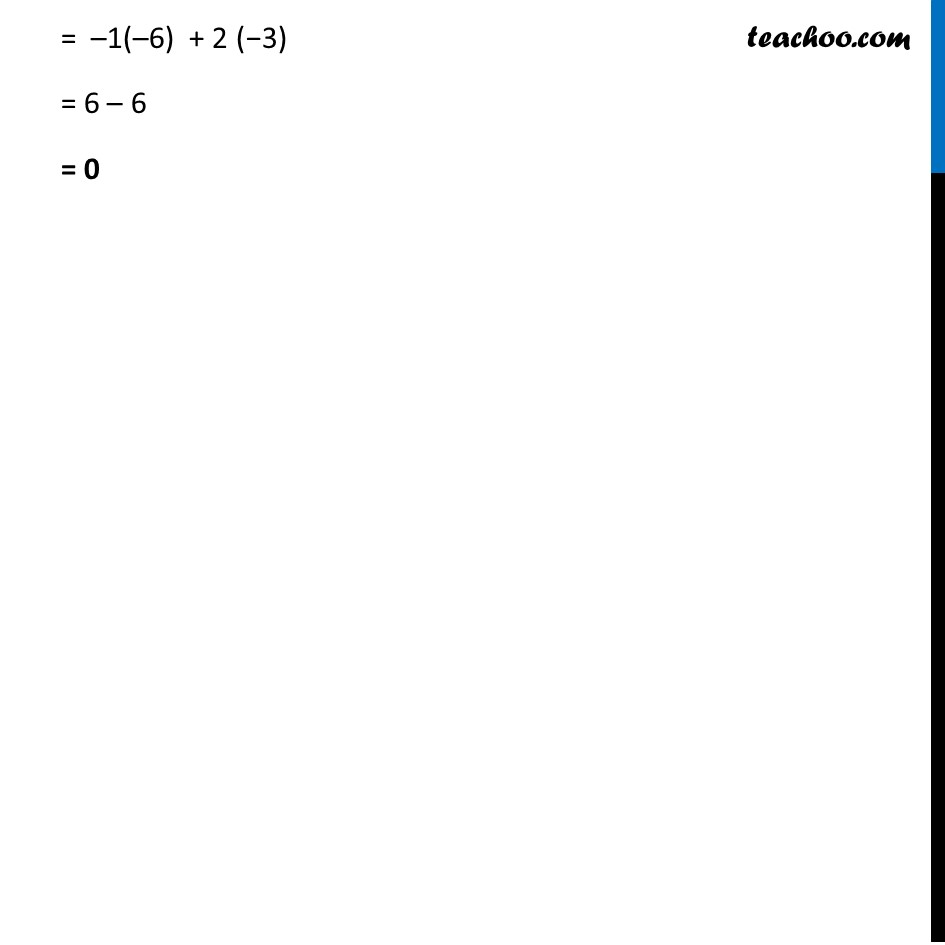Ex 4.1

Chapter 4 Class 12 Determinants
Serial order wiseLearn in your speed, with individual attention - Teachoo Maths 1-on-1 Class

### Transcript

Ex 4.1,5 Evaluate the determinants (iii) |■8(0&1&2@−1&0&−3@−2&3&0)| |■8(0&1&2@−1&0&−3@−2&3&0)| = 0 |■8(0&−3@3&0)| − 1 |■8(−1&−3@−2&0)| + 2 |■8(−1&0@−2&3)| = 0 (0(0)−3(−3))−1(−1(0)−(−2) (−3))+2 (−1(3)−(−2)0) = 0 (0 + 9) – 1 (0 – 6) +2 (– 3 – 0) = 0 – 1 (0 – 6) + 2 (−3 − 0) = –1(–6) + 2 (−3) = 6 – 6 = 0